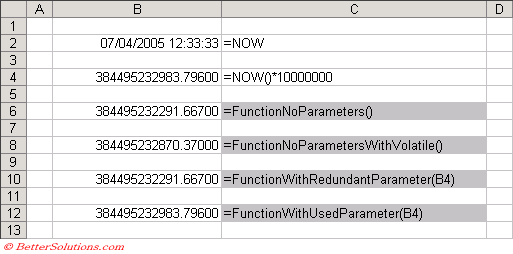### Recalculating

This is not as straight forward as you might think and depends on the following:
1) Which shortcut keys are pressed: F9, (Shift + F9), (Ctrl + Shift + F9), etc.
2) Whether any of the input parameters change.
3) Whether your custom function contains "Call Application.Volatile(True)".

#### Application.Volatile

If the following line of code is included in your user defined function then it will be recalculated every time a value changes on that particular worksheet.

`Call Application.Volatile(True) `

This may add a significant calculation overhead depending on how many times the function is used.
Passing in False causes the function to be recalculated only when one or more of its arguments change as a result of a recalculation.
This method is only relevant to functions that have arguments, any functions with no arguments will have to be manually updated ?? CHECK !!

#### Testing the Functions

We have written four simple custom worksheet functions to illustrate the differences.

`Public Function FunctionNoParameters() As String    FunctionNoParameters = CStr(Format(Now() * 10000000, "0.00000")) End Function Public Function FunctionNoParametersWithVolatile() As String    Call Application.Volatile(True)    FunctionNoParametersWithVolatile = CStr(Format(Now() * 10000000, "0.00000")) End Function Public Function FunctionWithRedundantParameter(ByVal rgeRange As Range) As String    FunctionWithRedundantParameter = CStr(Format(Now() * 10000000, "0.00000")) End Function Public Function FunctionWithUsedParameter(ByVal rgeRange As Range) As String    FunctionWithUsedParameter = CStr(Format(rgeRange.Value, "0.00000")) End Function `

#### Using the Functions

These custom functions are then referenced in the normal way from your worksheet.#### Pressing F9

Pressing F9 recalculates any cells that have changed in all the open workbooks.
This will only calculale formulas that have changed since the last calculation.
Only custom functions which contain the Application.Volatile statement or functions that have parameters which are referencing cells whose values change will be recalculated.
Any functions that use parameters to cell references must use the value in there calculation.

#### Pressing (Shift + F9)

Pressing (Shift + F9) is the same as pressing F9 except that it only recalculates cells on the active worksheet.
Only custom functions which contain the Application.Volatile statement or functions that have parameters which are referencing cells whose values change will be recalculated.
Any functions that use parameters to cell references must use the value in there calculation.

#### Pressing (Ctrl + Alt + F9)

Pressing (Ctrl + Alt + F9) recalculates all cells in all open workbooks regardless of whether they need to be recalculated.
This is often referred to as full calculation.
All custom functions will be recalculated regardless.

#### Pressing (Ctrl + Shift + F9)

Pressing (Ctrl + Shift + F9) does not seem to work in Excel 2003 or Excel 2002.
It is meant to recalculate all cells in the active workbook regardless of whether they need to be recalculated.

#### Pressing (Ctrl + Alt + Shift + F9)

Pressing (Ctrl + Alt + Shift + F9) recalculates all cells in all open workbooks regardless of whether they need to be recalculated.
This is often referred to a full calculation with dependency tree rebuild.
This includes all custom worksheet functions and external worksheet functions using a DDE.

#### Important

Pressing (Ctrl + Shift + F9) does not seem to work in Excel 2002 or Excel 2003.
Remember that the settings on the (Tools > Options)(Calculation tab) are workbook specific but it is the first workbook that is opened that determines what the settings are. Opening subsequent workbooks will not change the options.